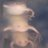## Influence of sea cucumbers on chromophoric of dissolved organic matter in multitrophic aquaculture tanks1320 days ago
Influence of sea cucumbers on chromophoric of dissolved organic matter in multitrophic aquaculture tanks https://t.co/2K2tqaMDix
NOT PEER-REVIEWED
"PeerJ Preprints" is a venue for early communication or feedback before peer review. Data may be preliminary.

A peer-reviewed article of this Preprint also exists.

View peer-reviewed version

## Supplemental Information

### CDOM and ancillary data of inlet water, tanks and effluents

Table S1. CDOM and ancillary data of inlet water. The a 325 values are the absorption coefficients at wavelength 325 nm (m-1), the S275-295 values are the spectral slopes at the wavelength band from 275 to 295 nm (μm-1), SR is the ratio of the slope between 275 and 295 nm divided by the slope between 350 and 400 nm (unitless) and the a*325 values are the molar absorption coefficients at wavelength 325 nm (mg C l-1m-1). TOC is total organic carbon in mg C l-1, POM is particulate organic carbon in mgl-1, chl a is the concentration of chlorophyll a in μg l-1 and BA is the bacterial abundance in (x106 cells ml-1). x-no datum

Table S2. CDOM and ancillary data of the +holothurian effluent water. The a 325 values are the absorption coefficients at wavelength 325 nm (m-1), the S275-295 values are the spectral slopes at the wavelength band from 275 to 295 nm (μm-1), SR is the ratio of the slope between 275 and 295 nm divided by the slope between 350 and 400 nm (unitless) and the a*325 values are the molar absorption coefficients at wavelength 325 nm (mg C l-1m-1). TOC is total organic carbon in mg C l-1, POM is particulate organic carbon in mgl-1, chl a is the concentration of chlorophyll a in μgl-1 and BA is the bacterial abundance in (x106 cells ml-1).

Table S3. CDOM and ancillary data of the -holothurian effluent water. The a 325values are the absorption coefficients at wavelength 325 nm (m-1), the S275-295 values are the spectral slopes at the wavelength band from 275 to 295 nm (μm-1), SR is the ratio of the slope between 275 and 295 nm divided by the slope between 350 and 400 nm (unitless) and the a*325values are the molar absorption coefficients at wavelength 325 nm (mg C l-1m-1). TOC is total organic carbon in mg C l-1, POM is particulate organic carbon in mgl-1, chl a is the concentration of chlorophyll a in μgl-1 and BA is the bacterial abundance in (x106 cells ml-1).

Table S4. CDOM and ancillary data of the +holothurian tank water. The a 325values are the absorption coefficients at wavelength 325 nm (m-1), the S275-295 values are the spectral slopes at the wavelength band from 275 to 295 nm (μm-1), SR is the ratio of the slope between 275 and 295 nm divided by the slope between 350 and 400 nm (unit less) and the a*325values are the molar absorption coefficients at wavelength 325 nm (mg C l-1m-1). TOC is total organic carbon in mg C l-1, POM is particulate organic carbon in mgl-1, chl a is the concentration of chlorophyll a in μgl-1 and BA is the bacterial abundance in (x106 cells ml-1).

Table S5. CDOM and ancillary data of the -holothurian tank water. The a 325values are the absorption coefficients at wavelength 325 nm (m-1), the S275-295 values are the spectral slopes at the wavelength band from 275 to 295 nm (μm-1), SR is the ratio of the slope between 275 and 295 nm divided by the slope between 350 and 400 nm (unit less) and the a*325values are the molar absorption coefficients at wavelength 325 nm (mg C l-1m-1). TOC is total organic carbon in mg C l-1, POM is particulate organic carbon in mgl-1, chl a is the concentration of chlorophyll a in μgl-1 and BA is the bacterial abundance in (x106 cells ml-1).

### Raw data of CDOM spectra

Raw data of CDOM spectra

### Competing Interests

The authors declare that they have no competing interests.

### Author Contributions

Seyed Mohammad Sadeghi-Nassaj performed the experiments, analyzed the data, contributed reagents/materials/analysis tools, wrote the paper, prepared figures and/or tables, reviewed drafts of the paper.

Teresa S. Catalá analyzed the data, reviewed drafts of the paper.

Pedro A. Álvarez conceived and designed the experiments, reviewed drafts of the paper, installations.

Isabel Reche conceived and designed the experiments, wrote the paper, prepared figures and/or tables, reviewed drafts of the paper.

### Funding

This work was financed by the Campus de Excelencia Internacional CEI BioTic Universidad de Granada, Spain grant PBS46 to IR and a PhD fellowship from the Iranian Ministry of Science to MS S-N. The funders had no role in study design, data collection and analysis, decision to publish, or preparation of the manuscript.

Some Markdown syntax is allowed: _italic_ **bold** ^superscript^ ~subscript~ %%blockquote%% [link text](link URL)